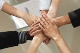# accumulate values in cell column

GridTop achievementsRank 1
Loyal User asked on 23 Dec 2013, 01:59 PM
i have a radgrid with columns:  "x" and "Total"
what i need is to display accumulated value in each row , e.i. :
first row contains x : 90 and total should be = 90+0;
second row contains : x= 15 and total should be : 15+ 90(previous value)= 105

etc ...

how can achieve that, by using itemDataBound event to set total value ,

## 2 Answers, 1 is accepted

0Eyup
Telerik teamanswered on 25 Dec 2013, 12:17 PM
Hi,

You can use a GridCalculatedColumn to achieve the requested functionality:
`<``telerik:GridCalculatedColumn ``UniqueName``=``"CalculatedColumnName"`
`    ``Expression``=``"{0}+{1}" ``DataFields``=``"OrderID,Freight"``>`
`</``telerik:GridCalculatedColumn``>`

Alternatively, you can access and calculate the values manually on the code-behind:
http://www.telerik.com/help/aspnet-ajax/grid-accessing-cells-and-rows.html

Hope this helps. Please give it a try and let me know if it works for you.

Regards,
Eyup
Telerik
If you want to get updates on new releases, tips and tricks and sneak peeks at our product labs directly from the developers working on the RadControls for ASP.NET AJAX, subscribe to the blog feed now.
0Princy
Top achievementsRank 2
answered on 26 Dec 2013, 10:39 AM
Hi ,

ASPX:
`<``telerik:GridBoundColumn` `DataField``=``"X"` `HeaderText``=``"X"` `UniqueName``=``"X"` `/>`
`<``telerik:GridTemplateColumn``>`
`    ``<``ItemTemplate``>`
`        ``<``asp:Label` `ID``=``"Label1"` `runat``=``"server"``></``asp:Label``>`
`    ``</``ItemTemplate``>`
`</``telerik:GridTemplateColumn``>`

C#:
`int` `sum = 0;`
`protected` `void` `RadGrid1_ItemDataBound(``object` `sender, GridItemEventArgs e)`
`{`
`    ``if` `(e.Item ``is` `GridDataItem)`
`    ``{`
`        ``GridDataItem item = (GridDataItem)e.Item;           `
`        ``int` `num1 = Convert.ToInt16(item[``"X"``].Text);`
`        ``Label total = (Label)item.FindControl(``"Label1"``);`
`        ``total.Text = num1.ToString();`
`        ``int` `index = item.ItemIndex;`
`        ``if` `(index > 1)`
`        ``{`
`            ``GridDataItem previous = (GridDataItem)RadGrid1.Items[index - 1];`
`            ``Label lbltotal = (Label)previous.FindControl(``"Label1"``);`
`            ``sum =Convert.ToInt16(lbltotal.Text);`
`            ``lbltotal.Text = (sum + num1).ToString();`
`        ``}`
`    ``}`
`}`

Thanks,
Princy
Tags
GridLoyal User
Top achievementsRank 1Rank 2